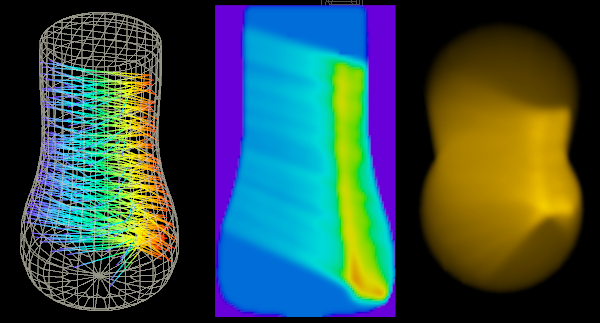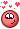# Volume Caustics

## Recommended Posts

I sampled refraction curves into a volume using SOPs and used the resulting density as emission inside a volume shader.Is there any way to realize volume caustics fully inside a material shader (either Mantra or Karma)?

volume_refraction.hipnc

•8
##### Share on other sites

• 4 months later...

I didn't see this Topic  interesting@konstantin magnus
https://github.com/miloyip/light2d

Edited by Librarian
•1
##### Share on other sites

• 2 years later...

Thank you Tesan!

I've just translated the first example from C to VEX for a volume wrangle.

```// Use Monte Carlo integration and ray marching
// of signed distance field (SDF) to render emissive circles.
// Source: https://github.com/miloyip/light2d/blob/master/basic.c

int samples = chi('samples');

function float sd_circle(vector2 uv, pos; float radius){
float dist = distance(uv, pos) - radius;
return dist;
}

function float sd_scene(vector2 uv){
float d_0 = sd_circle(uv, {0.3, 0.18}, 0.12);
float d_1 = sd_circle(uv, {0.25, 0.18}, 0.12);
float d_2 = sd_circle(uv, {-0.3, -0.2}, 0.02);
float dist = min( max(d_0, -d_1), d_2);
return max(dist);
}

function float trace(vector2 uv, dist){
float t = 0.0;
for(int i = 0; i < 100 && t < 2.0; i++){
float sd = sd_scene(uv + dist * t);
if(sd < 1e-5) return 1.5;
t += sd;
}
return 0.0;
}

function float sample(vector2 uv; int samples){
float sum = 0.0;
for(int i = 0; i < samples; i++){
float a = 2.0 * PI * ((rand(uv) + i) / float(samples));
sum += trace(uv, set(cos(a), sin(a)));
}
return sum / float(samples);
}

vector2 uv = set(v@P.x, v@P.y);

f@d = sample(uv, samples);```

•4

## Join the conversation

You can post now and register later. If you have an account, sign in now to post with your account.
Note: Your post will require moderator approval before it will be visible.×   Pasted as rich text.   Paste as plain text instead

Only 75 emoji are allowed.

×   Your previous content has been restored.   Clear editor

×   You cannot paste images directly. Upload or insert images from URL.

×
×